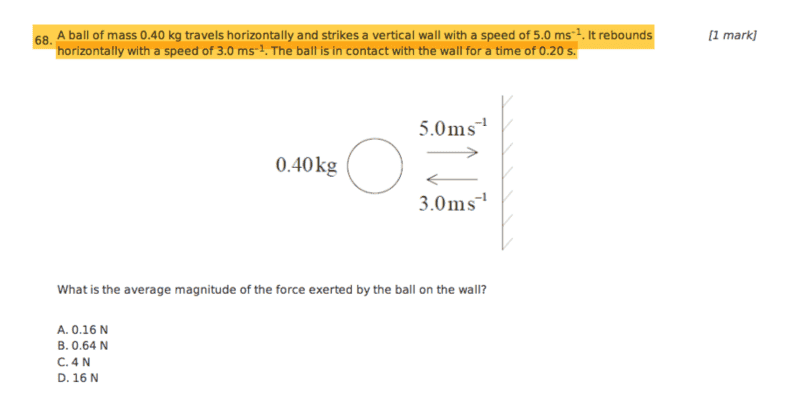# Multiple choice question

EF17xx

## Homework StatementFnet= Δp/Δt
p=mv

## The Attempt at a Solution

p (before) = 0.4 x 5 = 2
p (after) = 0.4 x 3 = 1.2
Δp = 0.8
Δt = 0.2
Fnet= Δp/Δt
0.8/0.2 = 4N

Correct answer in textbook is D, I do not understand why?